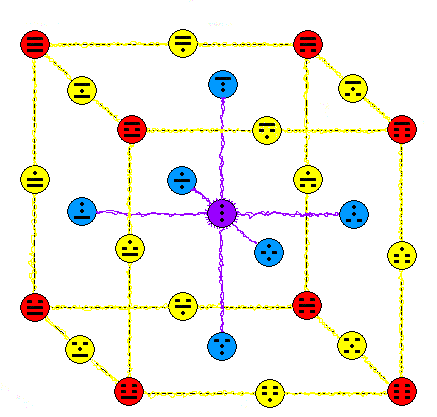About MeJames Barton

Independent truth seeker and webmaster of VirtueScience.com

Blogs Home » Browse Blogs » How Many Eternal Truths are there?

## How Many Eternal Truths are there?If we agree that there are eternal mathematical truths then the question arises how many are there..the answer will be either some finite number or infinite.

A sum such as 1+1=2 is an eternal truth and there are an infinite number of such sums.

If you study the diagram closely, it may take a while to appreciate it but there are 27 possible trinary permutations over 3 places.:Look closely:there are 27 geometric features of the cube: 8 corners, 12 edges, 6 faces and 1 center, 27 in total.

Of the 27 permutations there are exactly 8 with no 'dot' (in this case). There are exactly 12 permutations with one dot and there are exactly 6 with 2 dots. Then there is only one with all dots.

So we can see that interestingly there is a direct one to one correlation between the 27 permutations and the 27 geometric features of the cube.

Now the same relationship holds true for each of the infinite series of n-dimensional hypercubes with a corresponding set of trinary permutations.

There already we have 2 examples of infinite numbers of unchanging mathematical truths.

Do you have a different view on this? If we agree that there are eternal mathematical truths then the question arises how many are there..the answer will be either some finite number or infinite.

A sum such as 1+1=2 is an eternal truth and there are an infinite number of such sums.

If you study the diagram closely, it may take a while to appreciate it but there are 27 possible trinary permutations over 3 places.

Look closely: there are 27 geometric features of the cube: 8 corners, 12 edges, 6 faces and 1 center, 27 in total.

Of the 27 permutations there are exactly 8 with no 'dot' (in this case). There are exactly 12 permutations with one dot and there are exactly 6 with 2 dots. Then there is only one with all dots.

So we can see that interestingly there is a direct one to one correlation between the 27 permutations and the 27 geometric features of the cube.

Now the same relationship holds true for each of the infinite series of n-dimensional hypercubes with a corresponding set of trinary permutations.

There already we have 2 examples of infinite numbers of unchanging mathematical truths.

Do you have a different view on this?